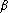# OEF RSA --- Introduction ---

This module actually contains 4 exercises on RSA cryptosystems: creation of an RSA system, encrypt/decrypt a message, cryptanalysis.

### Creation RSA

The objective of this exercise is to create an RSA cryptosystem (of simulation).

Step 1. Find two prime numbers, and , such that , . Compute .

You have taken , , .

Step 2. Find an integersuch that the map with is bijective on the set of invertible elements of . (You must take 10000.)

You have taken .

Step 3. Using as the public key of an RSA cryptosystem, what is the private key, that is, the integersuch that ?

### Encrypt RSA

The aim of this exercise is to simulate an RSA encryption by public key.

Suppose that in a secured communication, your partner has sent you a couple as the public key of an RSA cryptosystem, where

,
.

Now suppose that you have a message to sent to him. This (original) message is represented by the following number.

What is the corresponding crypted message that you should send? (The last number must be between 0 and N-1.)

### Decrypt RSA

The aim of this exercise is to decrypt a message on a given RSA cryptosystem (of simulation).

We have two prime numbers, and . Let

.

Take such that the couple forms the public key of an RSA cryptosystem.

Step 1. Compute the prive key, that is, the integersuch that xis the inverse of xin . (Among the choices of, you must take the smallest positive integer.)

Yes, .

Step 2. Using the public key , your partner has sent you the following crypted message (number). What is the original message (number)? (This must be a number between 0 and N-1.)

### Cryptanalysis RSA

You are listening a secured'' communication between two unknown people. One of them uses an RSA cryptosystem, with the public key

,
.

You notice that the key is too short, so that you can decrypt the messages without knowledge of the private key, by using the online tools on WIMS. You are tempted to do so.

Step 1. Compute the privatge key, that is, the integersuch that xis the inverse of xin . (Among the choices of, you should take the smallest positive integer.)

Yes, .

Step 2. Now you have intercepted the following crypted message (number). What is the original message (number)? (This must be a number between 0 and N-1.)

In order to access WIMS services, you need a browser supporting forms. In order to test the browser you are using, please type the word wims here: and press Enter''.